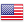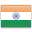Grade "A+" Accredited by NAAC with a CGPA of 3.46
Grade "A+" Accredited by NAAC with a CGPA of 3.46

# Quantitative Techniques

Course ID
FC 402
Level
Program
BBA (FIA)
Semester
Fourth
Credits
6.0
Paper Type
Core Course
Method
Lecture & Tutorial

## Unique Paper Code: 61011402

To apprise students with the construction of mathematical models for managerial decision situations and to use spreadsheets or computer software packages to obtain a solution wherever applicable. The emphasis is on understanding the concepts, formulation and interpretation.

## Learning Outcomes:

At the end of the course, students should be able to:

• Identify, formulate and solve Linear Programming Problems graphically, mathematically and by using excel solver.
• Solve optimization problems like transportation and assignment problem mathematically and by using excel solver.
• Develop critical thinking and use PERT and CPM techniques to improve decision making.
• Identify different types of decision-making environments and choose the appropriate decision-making approaches for each

## Course Contents

Unit I
Unit II
Unit III
Unit IV

Unit I (4 weeks)

Linear Programming
Formulation of L.P. Problems, Graphical Solutions (Special cases: Multiple optimal solution, infeasibility, unbounded solution); Simplex Method, Special cases, Big-M method and Two-phase method; Duality (emphasis on formulation & economic interpretation); Sensitivity Analysis; Formulation of Integer programming (Media Allocation Problem), Zero-one programming.

References:

Quantitative Techniques in Management, N. D. Vohra, 5th ed., Tata McGraw Hill. (Chapter 2, 3, 4 and 7)

Mohan Man, Gupta P. K., Swarup Kanti, Introduction to Management Science Operations Research, 19th ed. Sultan Chand & Sons. (Chapter 2, 3, 4, 5, 6 and 7)

Unit II (2 weeks)

Transportation and Assignment Problem
Transportation Problem: Formulation, Solution by N.W. Corner Rule, Least Cost method, Vogel’s Approximation Method (VAM), Modified Distribution Method; Special cases: Multiple Solutions, Maximization case, unbalanced case, prohibited routes.

Assignment Problem: Hungarian Method, Special cases: Multiple Solutions, Maximization case, Unbalanced case, Restrictions on assignment.

References:

Quantitative Techniques in Management, N. D. Vohra, 5th ed., Tata McGraw Hill. (Chapter 5 and 6)

Mohan Man, Gupta P. K., Swarup Kanti, Introduction to Management Science Operations Research, 19th ed. Sultan Chand & Sons. (Chapter 10 and 11)

Unit III (3 weeks)

Network Analysis
Basic Concept, Construction of the Network diagram, Critical Path Analysis, float and slack analysis (Total float, free float, independent float), probability consideration in PERT, Time-Cost optimization in Project.

References:

Quantitative Techniques in Management, N. D. Vohra, 5th ed., Tata McGraw Hill. (Chapter 14)

Mohan Man, Gupta P. K., Swarup Kanti, Introduction to Management Science Operations Research, 19th ed. Sultan Chand & Sons. (Chapter 24 and 25)

Unit IV (3 weeks)

Decision Theory
Decision making environment, Construction of Pay off Table, Opportunity Loss Table, Decision under uncertainty, Decision under Risk: EMV, EOL, EVPI.

Markov Chains: Markov Process, Transition Probability Matrix, Predicting Future Market Shares, Equilibrium Conditions, Limiting probabilities, Chapman Kolmogorov equation.

Decision under Conflict: Game Theory, Two-person Zero-Sum games, Maximin Minimax Principle, Games without Saddle point- Mixed strategy, Dominance Rule; Reduction of m x n game and solution of 2×2, 2 x s, and r x 2 cases by Graphical Method.

Simulation: Concept, Monte Carlo Simulation.

References:

Quantitative Techniques in Management, N. D. Vohra, 5th ed., Tata McGraw Hill. (Chapter 17, 15, 8 and 16)

Mohan Man, Gupta P. K., Swarup Kanti, Introduction to Management Science Operations Research, 19th ed. Sultan Chand & Sons. (Chapter 16, 15, 17 and 22)

#### Text Books

Quantitative Techniques in Management, N. D. Vohra,5th ed., Tata McGraw Hill.
Operations Research: An Introduction, 9th ed.,Hamdy A Taha, Pearson
MohanMan, GuptaP. K., SwarupKanti, Introduction to Management Science Operations Research, 19th ed. Sultan Chand & Sons.

Sharma J.K., Operations Research: Theory And Applications, 6th ed. Trinity.
Introduction to Operations Research, Frederick.S. Hiller, G.J. Liebermann, 10th ed.
Barry Render and T. N. Badri, Quantitative Analysis for Management, Pearson.

#### Teaching Learning Process

Lecture, Solving of numerical problems, discussion and PowerPoint presentations.

MS Excel solver or spreadsheet applications may also be demonstrated to students for LPP, Transportation and Assignment problems. Interface with project management, tora or other computer software packages may be provided to the students wherever applicable.

#### Assessment Methods

• Internal Assessment: 25 marks
• Written Theory Exam: 75 marks

#### Keywords

Linear programming, simplex method, transportation, assignment, network analysis, PERT, CPM, decision making, markov, game theory.

Disclaimer: Details on this page are subject to change as per University of Delhi guidelines. For latest update in this regard please refer to the University of Delhi website here.Englishहिन्दी